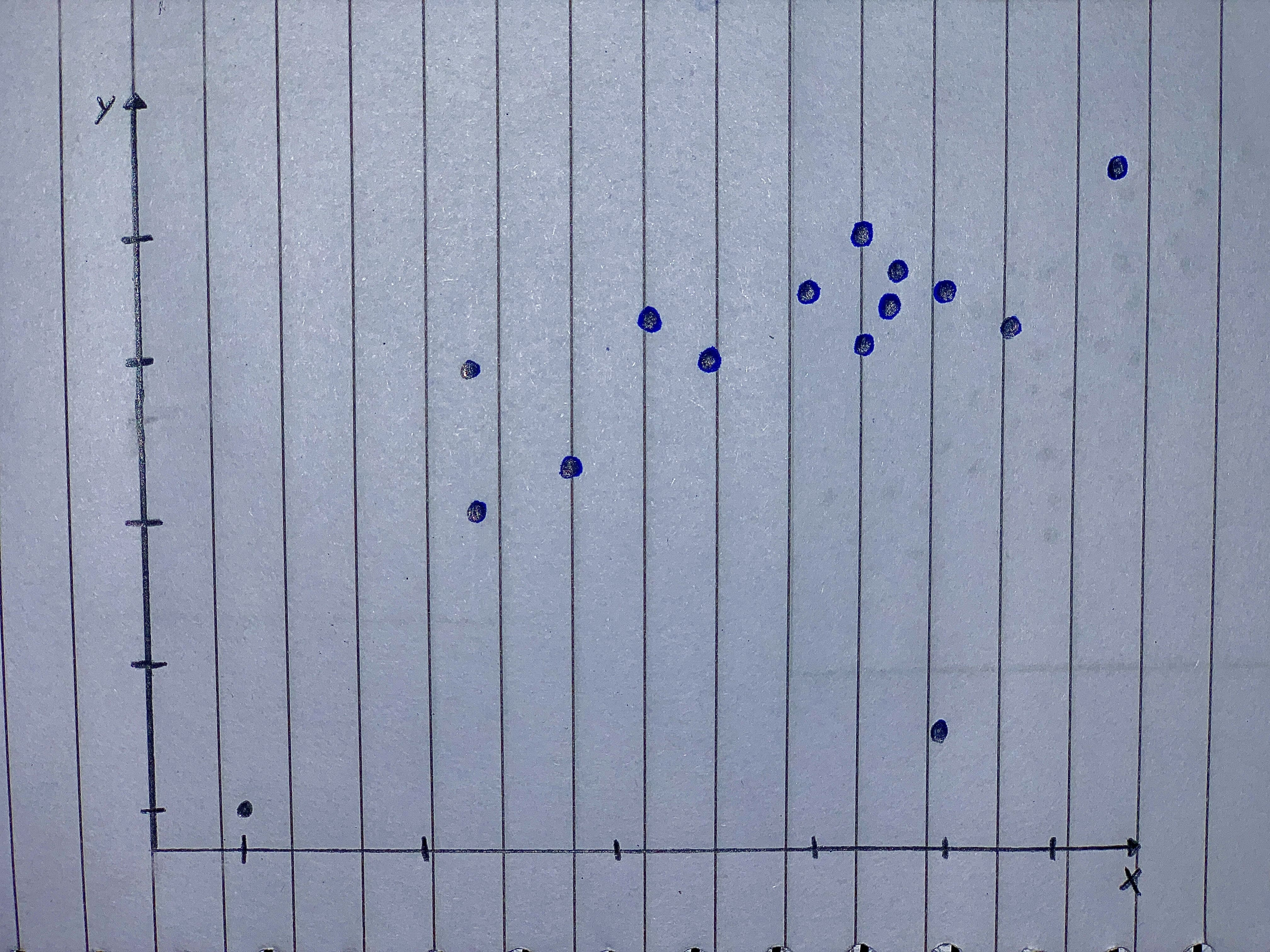Question# Straight Lines Graph the straight lines in Exercises 1–3.Then find the change in y for a one-uni

Scatterplots
ANSWEREDStraight Lines Graph the straight lines in Exercises 1–3.
Then find the change in y for a one-unit change in x, find the point at which the line crosses the y-axis, and calculate the value of y when x 52.5.
1. $$y x\ 5\ 1\ 2.0\ 0.5$$
2. $$y x\ 5\ 1\ 40\ 36.2$$
3. $$y x\ 5\ 25\ 6$$
Scatterplots For the scatterplots in Exercises 5 describe the pattern that you see. How strong is the pattern? Do you see any outliers or clusters?..........................2020-11-30
Step 1
From the given graph, it is observed that, as x increases y also increases so the direction of the graph is positive.
The given graph is linear as it does follow a straight line path with respect to two variables of graph.
Step 2
The strength of given graph is stronger as there are good number of samples along the line of curve.
There are two cluster of points at the bottom side of the graph where multiple points exist along the line. There is two outlier points as it appears out of the range of linear pattern in graph.